# allcycles

Find all cycles in graph

## Syntax

``cycles = allcycles(G)``
``[cycles,edgecycles] = allcycles(G)``
``[___] = allcycles(G,Name,Value)``

## Description

example

````cycles = allcycles(G)` returns all cycles in the specified graph. The output `cycles` is a cell array where the contents of each cell `cycles{k}` lists nodes that form a cycle.```

example

````[cycles,edgecycles] = allcycles(G)` also returns the edges in each cycle. The output `edgecycles` is a cell array where `edgecycles{k}` gives the edges in the corresponding cycle, `cycles{k}`.```

example

````[___] = allcycles(G,Name,Value)` specifies additional options using one or more name-value arguments. You can use any of the output argument combinations in previous syntaxes. For example, you can specify `MaxNumCycles` and a scalar to limit the number of cycles returned.```

## Examples

collapse all

Create a directed graph with nine nodes. Plot the graph.

```s = [1 2 3 6 5 5 4 6 9 8 8 7]; t = [2 3 6 5 2 4 1 9 8 5 7 4]; G = digraph(s,t); plot(G)```Calculate all cycles in the graph.

`cycles = allcycles(G)`
```cycles=5×1 cell array {[ 1 2 3 6 5 4]} {[1 2 3 6 9 8 5 4]} {[1 2 3 6 9 8 7 4]} {[ 2 3 6 5]} {[ 2 3 6 9 8 5]} ```

The second output argument of `allcycles` returns the edges that are contained in each cycle. This is particularly useful for multigraphs, where the edge index is required to uniquely identify the edges in each cycle.

Create a directed multigraph with eight nodes and 18 edges. Specify names for the nodes. Plot the graph with labeled nodes and edges.

```s = [1 1 2 2 3 3 2 2 4 6 8 6 6 7 3 3 5 3]; t = [2 3 1 3 2 1 4 4 6 2 6 7 8 8 5 5 7 7]; names = {'A','B','C','D','E','F','G','H'}; G = digraph(s,t,[],names); p = plot(G,'EdgeLabel',1:numedges(G));```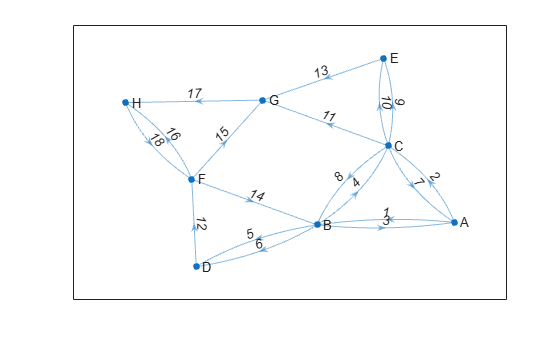Calculate all cycles in the graph. Specify two output arguments to also return the edge indices for edges in each cycle.

`[cycles,edgecycles] = allcycles(G);`

View the nodes and edges in the fifth cycle.

`cycles{5}`
```ans = 1x7 cell {'A'} {'C'} {'E'} {'G'} {'H'} {'F'} {'B'} ```
`edgecycles{5}`
```ans = 1×7 2 9 13 17 18 14 3 ```

Highlight the nodes and edges in the fifth cycle.

`highlight(p,'Edges',edgecycles{5},'EdgeColor','r','LineWidth',1.5,'NodeColor','r','MarkerSize',6)`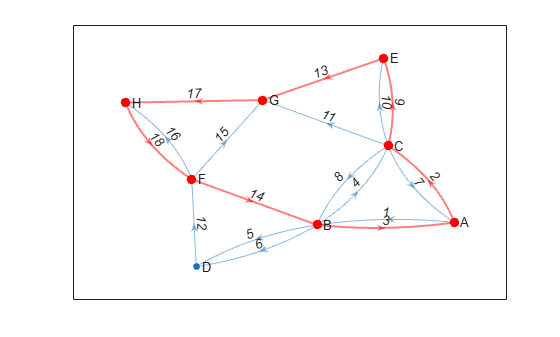Use the `'MaxNumCycles'`, `'MaxCycleLength'`, and `'MinCycleLength'` options to limit the number of cycles returned by `allcycles`.

Create an adjacency matrix for a complete graph with 20 nodes. Create an undirected graph from the adjacency matrix, omitting self-loops.

```A = ones(20); G = graph(A,'omitselfloops');```

Since all of the nodes in the graph are connected to all other nodes, there are a large number of cycles in the graph (more than `1.7e17`). Therefore, it is not feasible to calculate all of the cycles since the results will not fit in memory. Instead, calculate the first 10 cycles.

`cycles1 = allcycles(G,'MaxNumCycles',10)`
```cycles1=10×1 cell array {[ 1 2 3]} {[ 1 2 3 4]} {[ 1 2 3 4 5]} {[ 1 2 3 4 5 6]} {[ 1 2 3 4 5 6 7]} {[ 1 2 3 4 5 6 7 8]} {[ 1 2 3 4 5 6 7 8 9]} {[ 1 2 3 4 5 6 7 8 9 10]} {[ 1 2 3 4 5 6 7 8 9 10 11]} {[1 2 3 4 5 6 7 8 9 10 11 12]} ```

Now calculate the first 10 cycles that have a cycle length less than or equal to 3.

`cycles2 = allcycles(G,'MaxNumCycles',10,'MaxCycleLength',3)`
```cycles2=10×1 cell array {[ 1 2 3]} {[ 1 2 4]} {[ 1 2 5]} {[ 1 2 6]} {[ 1 2 7]} {[ 1 2 8]} {[ 1 2 9]} {[1 2 10]} {[1 2 11]} {[1 2 12]} ```

Finally, calculate the first 10 cycles that have a cycle length greater than or equal to 4.

`cycles3 = allcycles(G,'MaxNumCycles',10,'MinCycleLength',4)`
```cycles3=10×1 cell array {[ 1 2 3 4]} {[ 1 2 3 4 5]} {[ 1 2 3 4 5 6]} {[ 1 2 3 4 5 6 7]} {[ 1 2 3 4 5 6 7 8]} {[ 1 2 3 4 5 6 7 8 9]} {[ 1 2 3 4 5 6 7 8 9 10]} {[ 1 2 3 4 5 6 7 8 9 10 11]} {[ 1 2 3 4 5 6 7 8 9 10 11 12]} {[1 2 3 4 5 6 7 8 9 10 11 12 13]} ```

Examine how the outputs of the `cyclebasis` and `allcycles` functions scale with the number of edges in a graph.

Create and plot a square grid graph with three nodes on each side of the square.

```n = 5; A = delsq(numgrid('S',n)); G = graph(A,'omitselfloops'); plot(G)```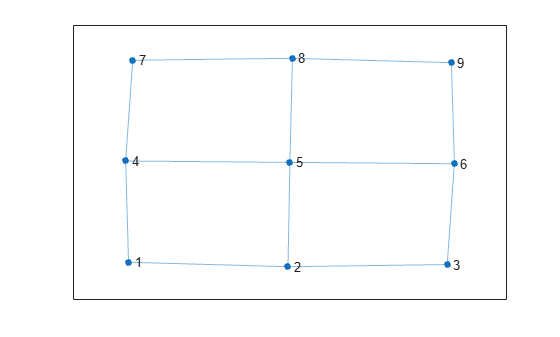Compute all cycles in the graph using `allcycles`. Use the `tiledlayout` function to construct an array of subplots and highlight each cycle in a subplot. The results indicate there are a total of 13 cycles in the graph.

```[cycles,edgecycles] = allcycles(G); tiledlayout flow for k = 1:length(cycles) nexttile highlight(plot(G),cycles{k},'Edges',edgecycles{k},'EdgeColor','r','NodeColor','r') title("Cycle " + k) end```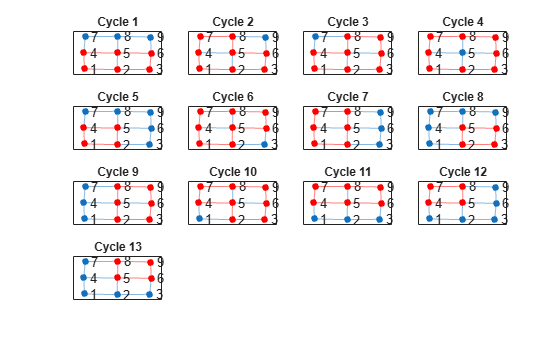Some of these cycles can be seen as combinations of smaller cycles. The `cyclebasis` function returns a subset of the cycles that form a basis for all other cycles in the graph. Use `cyclebasis` to compute the fundamental cycle basis and highlight each fundamental cycle in a subplot. Even though there are 13 cycles in the graph, there are only four fundamental cycles.

```[cycles,edgecycles] = cyclebasis(G); tiledlayout flow for k = 1:length(cycles) nexttile highlight(plot(G),cycles{k},'Edges',edgecycles{k},'EdgeColor','r','NodeColor','r') title("Cycle " + k) end```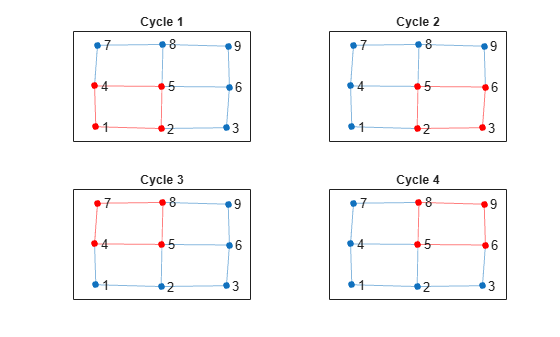Now, increase the number of nodes on each side of the square graph from three to four. This represents a small increase in the size of the graph.

```n = 6; A = delsq(numgrid('S',n)); G = graph(A,'omitselfloops'); figure plot(G)```Use `allcycles` to compute all of the cycles in the new graph. For this graph there are over 200 cycles, which is too many to plot.

`allcycles(G)`
```ans=213×1 cell array {[ 1 2 3 4 8 7 6 5]} {[ 1 2 3 4 8 7 6 10 9 5]} {[1 2 3 4 8 7 6 10 11 12 16 15 14 13 9 5]} {[ 1 2 3 4 8 7 6 10 11 15 14 13 9 5]} {[ 1 2 3 4 8 7 6 10 14 13 9 5]} {[ 1 2 3 4 8 7 11 10 6 5]} {[ 1 2 3 4 8 7 11 10 9 5]} {[ 1 2 3 4 8 7 11 10 14 13 9 5]} {[ 1 2 3 4 8 7 11 12 16 15 14 10 6 5]} {[ 1 2 3 4 8 7 11 12 16 15 14 10 9 5]} {[ 1 2 3 4 8 7 11 12 16 15 14 13 9 5]} {[1 2 3 4 8 7 11 12 16 15 14 13 9 10 6 5]} {[ 1 2 3 4 8 7 11 15 14 10 6 5]} {[ 1 2 3 4 8 7 11 15 14 10 9 5]} {[ 1 2 3 4 8 7 11 15 14 13 9 5]} {[ 1 2 3 4 8 7 11 15 14 13 9 10 6 5]} ⋮ ```

Despite the large number of cycles in the graph, `cyclebasis` still returns a small number of fundamental cycles. Each of the cycles in the graph can be constructed using only nine fundamental cycles.

```[cycles,edgecycles] = cyclebasis(G); figure tiledlayout flow for k = 1:length(cycles) nexttile highlight(plot(G),cycles{k},'Edges',edgecycles{k},'EdgeColor','r','NodeColor','r') title("Cycle " + k) end```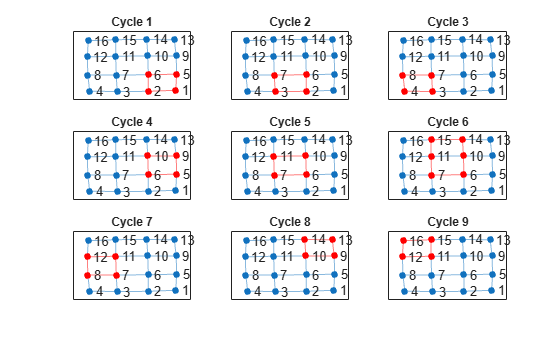The large increase in the number of cycles with only a small change in the size of the graph is typical for some graph structures. The number of cycles returned by `allcycles` can grow exponentially with the number of edges in the graph. However, the number of cycles returned by `cyclebasis` can, at most, grow linearly with the number of edges in the graph.

## Input Arguments

collapse all

Input graph, specified as either a `graph` or `digraph` object. Use `graph` to create an undirected graph or `digraph` to create a directed graph.

Example: `G = graph(1,2)`

Example: `G = digraph([1 2],[2 3])`

### Name-Value Arguments

Specify optional comma-separated pairs of `Name,Value` arguments. `Name` is the argument name and `Value` is the corresponding value. `Name` must appear inside quotes. You can specify several name and value pair arguments in any order as `Name1,Value1,...,NameN,ValueN`.

Example: `allcycles(G,'MaxNumCycles',100)` returns only the first 100 cycles in the graph.

Maximum number of cycles, specified as the comma-separated pair consisting of `'MaxNumCycles'` and a nonnegative integer scalar. This option is useful when the number of cycles in a graph grows large enough to hit memory limits. You can specify `MaxNumCycles` to limit the number of cycles returned by `allcycles` so that the results fit within available memory.

Example: `allcycles(G,'MaxNumCycles',100)`

Maximum cycle length, specified as the comma-separated pair consisting of `'MaxCycleLength'` and a positive integer scalar. This option filters the results returned by `allcycles` so that no cycles with length larger than the specified limit are returned. The length of a cycle is measured by the number of edges in it, ignoring edge weights.

To find cycles with a range of lengths, specify both `'MaxCycleLength'` and `'MinCycleLength'`. To find cycles with an exact specified length, specify the same value for both `'MaxCycleLength'` and `'MinCycleLength'`.

Example: `allcycles(G,'MaxCycleLength',4)` returns cycles that have a length less than or equal to 4.

Example: `allcycles(G,'MinCycleLength',3,'MaxCycleLength',5)` returns cycles that have a length of 3, 4, or 5.

Minimum cycle length, specified as the comma-separated pair consisting of `'MinCycleLength'` and a positive integer scalar. This option filters the results returned by `allcycles` so that no cycles with length smaller than the specified limit are returned. The length of a cycle is measured by the number of edges in it, ignoring edge weights.

To find cycles with a range of lengths, specify both `'MaxCycleLength'` and `'MinCycleLength'`. To find cycles with an exact specified length, specify the same value for both `'MaxCycleLength'` and `'MinCycleLength'`.

Example: `allcycles(G,'MinCycleLength',2)` returns cycles that have a length greater than or equal to 2.

Example: `allcycles(G,'MinCycleLength',3,'MaxCycleLength',5)` returns cycles that have a length of 3, 4, or 5.

## Output Arguments

collapse all

Graph cycles, returned as a cell array. Each element `cycles{k}` contains the nodes that belong to one of the cycles in `G`. Each cycle begins with the node that has the smallest node index, and the cycles are returned in lexicographical order. Cycles in undirected graphs are returned only once, following a single direction. If `G` does not contain any cycles, then `cycles` is empty.

The data type of the cells in `cycles` depends on whether the input graph contains node names:

• If graph `G` does not have node names, then each element `cycles{k}` is a numeric vector of node indices.

• If graph `G` has node names, then each element `cycles{k}` is a cell array of character vector node names.

Edges in each cycle, returned as a cell array. Each element `edgecycles{k}` contains the edge indices for edges in the corresponding cycle, `cycles{k}`. If `G` does not contain any cycles, then `edgecycles` is empty.

collapse all

### Graph Cycles

A cycle exists in a graph when there is a nonempty path in which only the first and last nodes are repeated. An example of a cycle is: (Node1 - Node2 - Node3 - Node1). By convention, `allcycles` does not return the last node in the cycle since it is the same as the first.

A cycle cannot traverse the same edge twice. For example, the cycle (Node1 - Node2 - Node1) in an undirected graph only exists if there is more than one edge connecting Node1 and Node2. By this definition, self-loops count as cycles, though they cannot be part of a larger cycle.

## Tips

• The number of cycles in a graph depends heavily on the structure of the graph. For some graph structures, the number of cycles can grow exponentially with the number of nodes. For example, a complete graph with 12 nodes given by ```G = graph(ones(12))``` contains nearly 60 million cycles. Use the `MaxNumCycles`, `MaxCycleLength`, and `MinCycleLength` options to control the output of `allcycles` in these cases.